# The Azimuth Project Blog - network theory (part 24)

This page is a blog article in progress, written by John Baez. To see discussions of this article while it was being written, go to the Azimuth Forum. For the final polished version, go to the Azimuth Blog.

Now we’ve reached the climax of our story so far: we’re ready to prove the deficiency zero theorem. First let’s talk about it informally a bit. Then we’ll review the notation, and then—hang on to your seat!—we’ll give the proof.

The crucial trick is to relate a bunch of chemical reactions, described by a ‘reaction network’ like this: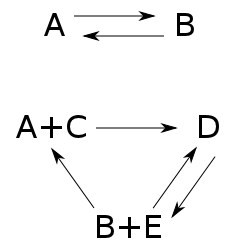to a simpler problem where a system randomly hops between states arranged in the same pattern: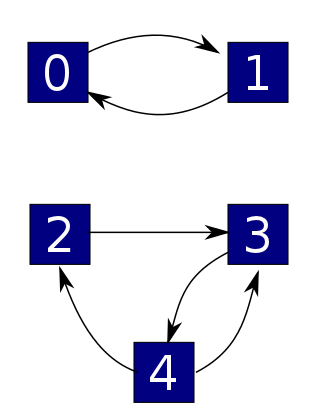This is sort of amazing, because we’ve thrown out lots of detail. It’s also amazing because this simpler problem is linear. In the original problem, the chance that a reaction turns a B + E into a D is proportional to the number of B’s times the number of E’s. That’s nonlinear! But in the simplified problem, the chance that your system hops from state 4 to state 3 is just proportional to the probability that it’s in state 4 to begin with. That’s linear.

The wonderful thing is that, at least under some conditions, we can an find an equilibrium for our chemical reactions starting from an equilibrium solution of the simpler problem.

Let’s roughly sketch how it works, and where we are so far. Our simplified problem is described by an equation like this:

$\displaystyle{ \frac{d}{d t} \psi = H \psi }$

where $\psi$ is a function that the probability of being in each state, and $H$ describes the probability per time of hopping from one state to another. We can easily understand quite a lot about the equilibrium solutions, where $\psi$ doesn’t change at all:

$H \psi = 0$

because this is a linear equation. We did this in Part 23. Of course, when I say ‘easily’, that’s a relative thing: we needed to use the Perron–Frobenius theorem, which Jacob introduced in Part 20. But that’s a well-known theorem in linear algebra, and it’s easy to apply here.

In Part 22, we saw that the original problem was described by an equation like this, called the ‘rate equation’:

$\displaystyle{ \frac{d x}{d t} = Y H x^Y }$

Here $x$ is a vector whose entries describe the amount of each kind of chemical: the amount of A’s, the amount of B’s, and so on. The matrix $H$ is the same as in the simplified problem, but $Y$ is a matrix that says how many times each chemical shows up in each spot in our reaction network:The key thing to notice is $x^Y$, where we take a vector and raise it to the power of a matrix. We explained this operation back in Part 22. It’s this operation that says how many B + E pairs we have, for example, given the number of B’s and the number of E’s. It’s this that makes the rate equation nonlinear.

Now, we’re looking for equilibrium solutions of the rate equation, where the rate of change is zero:

$Y H x^Y = 0$

But in fact we’ll do even better! We’ll find solutions of this:

$H x^Y = 0$

And we’ll get these by taking our solutions of this:

$H \psi = 0$

$\psi = x^Y$

while $\psi$ remains a solution of $H \psi = 0$.

But: how do we do this ‘adjusting’? That’s the crux of the whole business! That’s what we’ll do today.

Remember, $\psi$ is a function that gives a probability for each ‘state’, or numbered box here:The picture here consists of two pieces, called ‘connected components’: the piece containing boxes 0 and 1, and the piece containing boxes 2, 3 and 4. It turns out that we can multiply $\psi$ by a function that’s constant on each connected component, and if we had $H \psi = 0$ to begin with, that will still be true afterward. The reason is that there’s no way for $\psi$ to ‘leak across’ from one component to another. It’s like having water in separate buckets. You can increase the amount of water in one bucket, and decrease it another, and as long as the water’s surface remains flat in each bucket, the whole situation remains in equilibrium.

That’s sort of obvious. What’s not obvious is that we can adjust $\psi$ this way so as to ensure

$\psi = x^Y$

for some $x$.

And indeed, it’s not always true! It’s only true if our reaction network obeys a special condition. It needs to have ‘deficiency zero’. We defined this concept back in Part 21, but now we’ll finally use it for something. It turns out to be precisely the right condition to guarantee we can tweak any function on our set of states, multiplying it by a function that’s constant on each connected component, and get a new function $\psi$ with

$\psi = x^Y$

When all is said and done, that is the key to the deficiency zero theorem.

### Review

The battle is almost upon us—we’ve got one last chance to review our notation. We start with a stochastic reaction network: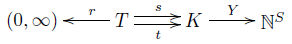This consists of:

• finite sets of transitions $T$, complexes $K$ and species $S$,

• a map $r: T \to (0,\infty)$ giving a rate constant for each transition,

source and target maps $s,t : T \to K$ saying where each transition starts and ends,

• a one-to-one map $Y : K \to \mathbb{N}^S$ saying how each complex is made of species.

Then we extend $s, t$ and $Y$ to linear maps: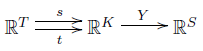Then we put inner products on these vector spaces as described last time, which lets us ‘turn around’ the maps $s$ and $t$ by taking their adjoints:

$s^\dagger, t^\dagger : \mathbb{R}^K \to \mathbb{R}^T$

More surprisingly, we can ‘turn around’ $Y$ and get a nonlinear map using ‘matrix exponentiation’:

$\begin{array}{ccc} \mathbb{R}^S &\to& \mathbb{R}^K \\ x &\mapsto& x^Y \end{array}$

This is most easily understood by thinking of $x$ as a row vector and $Y$ as a matrix:

$\begin{array}{ccl} x^Y &=& {\left( \begin{array}{cccc} x_1 , & x_2 , & \dots, & x_k \end{array} \right)}^{ \left( \begin{array}{cccc} Y_{11} & Y_{12} & \cdots & Y_{1 \ell} \\ Y_{21} & Y_{22} & \cdots & Y_{2 \ell} \\ \vdots & \vdots & \ddots & \vdots \\ Y_{k1} & Y_{k2} & \cdots & Y_{k \ell} \end{array} \right)} \\ \\ &=& \left( x_1^{Y_{11}} \cdots x_k^{Y_{k1}} ,\; \dots, \; x_1^{Y_{1 \ell}} \cdots x_k^{Y_{k \ell}} \right) \end{array}$

Remember, complexes are made out of species. The matrix entry $Y_{i j}$ says how many things of the $j$th species there are in a complex of the $i$th kind. If $\psi \in \mathbb{R}^K$ says how many complexes there are of each kind, $Y \psi \in \mathbb{R}^S$ says how many things there are of each species. Conversely, if $x \in \mathbb{R}^S$ says how many things there are of each species, $x^Y \in \mathbb{R}^K$ says how many ways we can build each kind of complex from them.

So, we get these maps: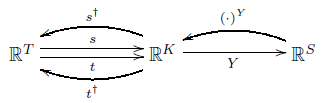Next, the boundary operator

$\partial : \mathbb{R}^T \to \mathbb{R}^K$

describes how each transition causes a change in complexes:

$\partial = t - s$

As we saw last time, there is a Hamiltonian

$H : \mathbb{R}^K \to \mathbb{R}^K$

describing a Markov processes on the set of complexes, given by

$H = \partial s^\dagger$

But the star of the show is the rate equation. This describes how the number of things of each species changes with time. We write these numbers in a list and get a vector $x \in \mathbb{R}^S$ with nonnegative components. The rate equation says:

$\displaystyle{ \frac{d x}{d t} = Y H x^Y }$

We can read this as follows:

$x$ says how many things of each species we have now.

$x^Y$ says how many complexes of each kind we can build from these species.

$s^\dagger x^Y$ says how many transitions of each kind can originate starting from these complexes, with each transition weighted by its rate.

$H x^Y = \partial s^\dagger x^Y$ is the rate of change of the number of complexes of each kind, due to these transitions.

$Y H x^Y$ is the rate of change of the number of things of each species.

### The zero deficiency theorem

We are looking for equilibrium solutions of the rate equation, where the number of things of each species doesn’t change at all:

$Y H x^Y = 0$

In fact we will find complex balanced equilibrium solutions, where even the number of complexes of each kind doesn’t change:

$H x^Y = 0$

More precisely, we have:

Deficiency Zero Theorem (Child’s Version). Suppose we have a reaction network obeying these two conditions:

1. It is weakly reversible, meaning that whenever there’s a transition from one complex $\kappa$ to another $\kappa'$, there’s a directed path of transitions going back from $\kappa'$ to $\kappa$.

2. It has deficiency zero, meaning $\mathrm{im} \partial \cap \mathrm{ker} Y = \{ 0 \}$.

Then for any choice of rate constants there exists a complex balanced equilibrium solution of the rate equation where all species are present in nonzero amounts. In other words, there exists $x \in \mathbb{R}^S$ with all components positive and such that:

$H x^Y = 0$

Proof. Because our reaction network is weakly reversible, the theorems in Part 23 show there exists $\psi \in (0,\infty)^K$ with

$H \psi = 0$

This $\psi$ may not be of the form $x^Y$, but we shall adjust $\psi$ so that it becomes of this form, while still remaining a solution of $H \psi = 0$. To do this, we need a couple of lemmas:

Lemma 1. $\mathrm{ker} \partial^\dagger + \mathrm{im} Y^\dagger = \mathbb{R}^K$.

Proof. We need to use a few facts from linear algebra. If $V$ is a finite-dimensional vector space with inner product, the orthogonal complement $L^\perp$ of a subspace $L \subseteq V$ consists of vectors that are orthogonal to everything in $L$:

$L^\perp = \{ v \in V : \quad \forall w \in L \quad \langle v, w \rangle = 0 \}$

We have

$(L \cap M)^\perp = L^\perp + M^\perp$

where $L$ and $M$ are subspaces of $V$ and $+$ denotes the sum of two subspaces: that is, the smallest subspace containing both. Also, if $T: V \to W$ is a linear map between finite-dimensional vector spaces with inner product, we have

$(\mathrm{ker} T)^\perp = \mathrm{im} T^\dagger$

and

$(\mathrm{im} T)^\perp = \mathrm{ker} T^\dagger$

Now, because our reaction network has deficiency zero, we know that

$\mathrm{im} \partial \cap \mathrm{ker} Y = \{ 0 \}$

Taking the orthogonal complement of both sides, we get

$(\mathrm{im} \partial \cap \mathrm{ker} Y)^\perp = \mathbb{R}^K$

and using the rules we mentioned, we obtain

$\mathrm{ker} \partial^\dagger + \mathrm{im} Y^\dagger = \mathbb{R}^K$

as desired.   █

Now, given a vector $\phi$ in $\mathbb{R}^K$ or $\mathbb{R}^S$ with all positive components, we can define the logarithm of such a vector, componentwise:

$(\ln \phi)_i = \ln (\phi_i)$

Similarly, for any vector $\phi$ in either of these spaces, we can define its exponential in a componentwise way:

$(\exp \phi)_i = \exp(\phi_i)$

These operations are inverse to each other. Moreover:

Lemma 2. The nonlinear operator

$\begin{array}{ccc} \mathbb{R}^S &\to& \mathbb{R}^K \\ x &\mapsto& x^Y \end{array}$

is related to the linear operator

$\begin{array}{ccc} \mathbb{R}^S &\to& \mathbb{R}^K \\ x &\mapsto& Y^\dagger x \end{array}$

by the formula

$x^Y = \exp(Y^\dagger \ln x )$

which holds for all $x \in (0,\infty)^S$.

Proof. A straightforward calculation. By the way, this formula would look a bit nicer if we treated $\ln x$ as a row vector and multiplied it on the right by $Y$: then we would have

$x^Y = \exp((\ln x) Y)$

The problem is that we are following the usual convention of multiplying vectors by matrices on the left, yet writing the matrix on the right in $x^Y$. Taking the transpose $Y^\dagger$ of the matrix $Y$ serves to compensate for this.   █

Now, given our vector $\psi \in (0,\infty)^K$ with $H \psi = 0$, we can take its logarithm and get $\ln \psi \in \mathbb{R}^K$. Lemma 1 says that

$\mathbb{R}^K = \mathrm{ker} \partial^\dagger + \mathrm{im} Y^\dagger$

so we can write

$\ln \psi = \alpha + Y^\dagger \beta$

where $\alpha \in \mathrm{ker} \partial^\dagger$ and $\beta \in \mathbb{R}^S$. Moreover, we can write

$\beta = \ln x$

for some $x \in (0,\infty)^S$, so that

$\ln \psi = \alpha + Y^\dagger (\ln x)$

Exponentiating both sides componentwise, we get

$\psi = \exp(\alpha) \; \exp(Y^\dagger (\ln x))$

where at right we are taking the componentwise product of vectors. Thanks to Lemma 2, we conclude that

$\psi = \exp(\alpha) x^Y$

So, we have taken $\psi$ and almost written it in the form $x^Y$—but not quite! We can adjust $\psi$ to make it be of this form:

$\exp(-\alpha) \psi = x^Y$

Clearly all the components of $\exp(-\alpha) \psi$ are positive, since the same is true for both $\psi$ and $\exp(-\alpha)$. So, the only remaining task is to check that

$H(\exp(-\alpha) \psi) = 0$

We do this using two lemmas:

Lemma 3. If $H \psi = 0$ and $\alpha \in \mathrm{ker} \partial^\dagger$, then $H(\exp(-\alpha) \psi) = 0$.

Proof. It is enough to check that multiplication by $\exp(-\alpha)$ commutes with the Hamiltonian $H$, since then

$H(\exp(-\alpha) \psi) = \exp(-\alpha) H \psi = 0$

Recall from Part 23 that $H$ is the Hamiltonian of a Markov process associated to this ‘graph with rates’: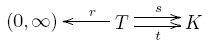As noted here:

• John Baez and Brendan Fong, A Noether theorem for Markov processes.

multiplication by some function on $K$ commutes with $H$ if and only if that function is constant on each connected component of this graph. Such functions are called conserved quantities.

So, it suffices to show that $\exp(-\alpha)$ is constant on each connected component. For this, it is enough to show that $\alpha$ itself is constant on each connected component. But this will follow from the next lemma, since $\alpha \in \mathrm{ker} \partial^\dagger$.   █

Lemma 4. A function $\alpha \in \mathbb{R}^K$ is a conserved quantity iff $\partial^\dagger \alpha = 0$. In other words, $\alpha$ is constant on each connected component of the graph $s, t: T \to K$ iff $\partial^\dagger \alpha = 0$.

Proof. Suppose $\partial^\dagger \alpha = 0$, or in other words, $\alpha \in \mathrm{ker} \partial^\dagger$, or in still other words, $\alpha \in (\mathrm{im} \partial)^\perp$. To show that $\alpha$ is constant on each connected component, it suffices to show that whenever we have two complexes connected by a transition, like this:

$\tau: \kappa \to \kappa'$

then $\alpha$ takes the same value at both these complexes:

$\alpha_\kappa = \alpha_{\kappa'}$

To see this, note

$\partial \tau = t(\tau) - s(\tau) = \kappa' - \kappa$

and since $\alpha \in (\mathrm{im} \partial)^\perp$, we conclude

$\langle \alpha, \kappa' - \kappa \rangle = 0$

But calculating this inner product, we see

$\alpha_{\kappa'} - \alpha_{\kappa} = 0$

as desired.

For the converse, we simply turn the argument around: if $\alpha$ is constant on each connected component, we see $\langle \alpha, \kappa' - \kappa \rangle = 0$ whenever there is a transition $\tau : \kappa \to \kappa'$. It follows that $\langle \alpha, \partial \tau \rangle = 0$ for every transition $\tau$, so $\alpha \in (\mathrm{im} \partial)^\perp$.

And thus concludes the proof of the lemma!   █

And thus concludes the proof of the theorem!   █

And thus concludes this post!

category: blog